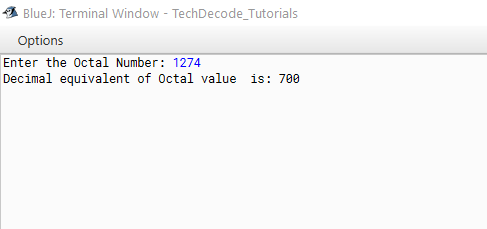# How to convert Octal to Decimal in Java

The Conversion Of Octal to Decimal in Java is the conversion of number bases. Let us know about both number types before we start.

This is an eight-base system that employs numbers ranging from 0 to 7. Apart from Binary, Decimal, and Hexadecimal Numbers, it is one of the classes of number systems. The octal symbol is used to represent numbers with an eight-digit base. The relevance and uses of octal numbers are numerous. One of the most popular applications is in computer fundamentals. An Octal number system has an ‘eight’ as its foundation and employs numbers ranging from 0 to 7, i.e., 0, 1, 2, 3, 4, 5, 6, and 7. To further explain the notion, we may use an example. Any number with a base of eight, such as 24(8), 109(8), or 55(8), is known as an octal number.

Decimal is a term that describes the base-10 number system, probably the most commonly used number system. The decimal number system consists of ten single-digit numbers: 0, 1, 2, 3, 4, 5, 6, 7, 8, and 9. The number after 9 is 10.

## What’s the Approach?

• Import `java.util` package into the class.

• Create a `Scanner Class` Object using `Scanner sc=new Scanner (System.in);`

• Accept user’s input and store it in a `string variable named oct.`

• Convert it to decimal using `Integer.parseInt(oct,8);`

• Print the `Decimal` value.

Also Read: How To Convert String To Boolean in Java

### Java Program to Convert Octal to Decimal:

```/*

* TechDecode Tutorials

*

* How to Convert Octal to Decimal

*

*/

import java.util.*;

public class Oct_To_Dec

{

public static void main(String args[])

{

Scanner sc=new Scanner(System.in);

//Accepting users input

System.out.print("Enter the Octal Number: ");

String oct = sc.nextLine();

//octal to decimal using Integer.parseInt()

int dec = Integer.parseInt(oct, 8);

System.out.println("Decimal equivalent of Octal value  is: "+dec);

}

}```

Output: